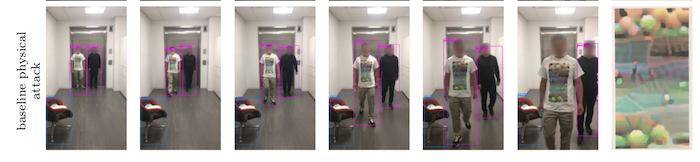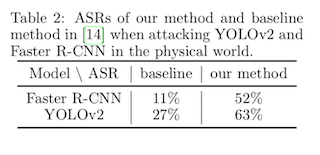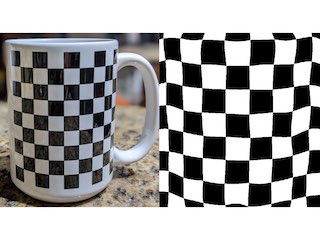In Evading real-time person detectors by adversarial t-shirt1, Xu and coauthors show that the adversarial patch attack described by Thys, Van Ranst, and Goedemé2 is less successful when applied to flexible media like fabric, due to the warping and folding that occurs.They propose to remedy this failure case by explicitly modeling the warping that occurs to a t-shirt when worn on a human torso, and applying those transformations during optimization of the adversarial patch. They argue that this elevates the real-world attack success rate from around 25% to a bit more than 50%, where success is defined as the failure of an object detection model to correctly label a human wearing the adversarial patch in a given image.The authors of the adversarial t-shirt paper, unlike the authors of the adversarial patch paper, have not released the code used to generate the thin plate spline function parameters used to warp the patch, the function parameters themselves, nor the code used to apply the transformation during the optimization routine.

There is a repository online with example code for applying thin plate splines3, but it does not appear to be associated with the authors of the adversarial t-shirt paper. The code itself appears visually to have the correct behavior, e.g. when used to learn the convex warp for a patch applied to a coffee mug:but is implemented in a non-optimized fashion. The relevant section of the repository looks like this:

``````new_img = np.zeros_like(orig_img)

for x in range(orig_img.shape):
for y in range(orig_img.shape):
new_x = int(round(x + spline_fn(x, y, *delta_x_args)))
new_y = int(round(y + spline_fn(x, y, *delta_y_args)))

if new_x >= 0 and new_x < orig_img.shape and new_y > 0 and new_y < orig_img.shape:
new_img[y, x, :] = orig_img[new_y, new_x, :]
``````

where the spline function looks like this:

``````def spline_fn(x, y, a, p, w):
p_dists = np.linalg.norm(p - np.array([x, y]), axis=1)
return np.dot(np.array([1, x, y]), a) + np.dot(w, u(p_dists))
``````

If we time how long it takes to execute this function on a single patch, we see that timeit reports the following:

``````1min 42s ± 8.63 s per loop (mean ± std. dev. of 7 runs, 1 loop each)
``````

In this particular example, the slow runtime is caused by the following:

1. `for` loops in Python have much more overhead than loops in C; and,
2. mathematical operations in Python involve several layers of type checks, safety checks, and translation between the Python and underlying C data types; and,
3. operating on a single pixel at a time destroys the advantages of data locality in memory.

The solution to these is to translate the looping logic to use vectorized operations from numpy, which are implemented in an optimized fashion in the C layer. One approach to vectorizing this logic is to initialize an array with all the x and y values, and operate on them simultaneously. That might look something like this:

``````    h = orig_img.shape[-3]
w = orig_img.shape[-2]
new_img = np.zeros_like(orig_img)

positions = np.stack([
np.ones((h, w)),
np.tile(np.arange(w), (h, 1)),
np.tile(np.arange(h), (w, 1)).T
])

new_x = (np.round(positions[1, :, :] + fast_spline_fn(positions, *delta_x_args)))
new_y = (np.round(positions[2, :, :] + fast_spline_fn(positions, *delta_y_args)))

positions = positions.astype('int64')
new_x = np.where((new_x < 0) | (new_x > w), positions, new_x).astype('int64')
new_y = np.where((new_y < 0) | (new_y > h), positions, new_y).astype('int64')
new_img[positions[2, :, :].ravel(), positions[1, :, :].ravel(), :] = orig_img[new_y.ravel(), new_x.ravel(), :]
``````

We'll also need to update the spline function, so that it is using matrix operations on the tensor of xy position integers, instead of operating on a single (x, y) tuple:

``````def fast_spline_fn(xy, a, p, w):
d = np.linalg.norm(p[..., None, None] - xy[1:], axis=1)
d = d * d * np.log(d, where=d!=0)
p = (xy.T @ a).T + w @ d.transpose(1, 0, 2)
return p
``````

After applying the changes, we can do a quick speed check for the new logic

``````4.97 ms ± 92.3 µs per loop (mean ± std. dev. of 7 runs, 100 loops each)
``````

where we see that rewriting the Python logic to use NumPy's vectorized operations has reduced the average runtime of this function call by about four orders of magnitude: to say this another way, the new logic is 10,000 times faster.